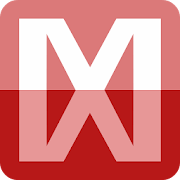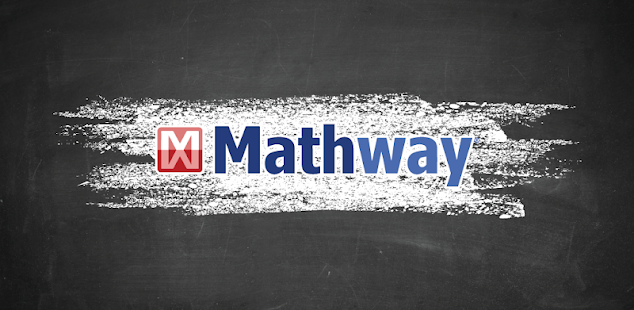Mathway: Scan Photos, Solve ProblemsEveryone
365,897
·
Offers in-app purchasesMathway is the world’s smartest math calculator for algebra, graphing, calculus and more! Simply point your camera and snap a photo or type your math homework question for step-by-step answers.

With millions of users and billions of problems solved, Mathway is like a private tutor in the palm of your hand, providing instant homework help anywhere, anytime.

Mathway covers all levels of math, including:

Basic Math/Pre-Algebra (arithmetic, integers, fractions, decimal numbers, roots, factors, and more...)

Algebra (linear equations/inequalities, quadratic equations/inequalities, absolute equations/inequalities, systems of equations, logarithms, functions, matrices, graphing, and more...)

Trigonometry/Precalculus (trigonometric functions, identities, conic sections, vectors, matrices, complex numbers, sequences and series, and more...)

Calculus (limits, derivatives, integrals, and more...)

Statistics (probability, permutations, combinations, and more...)

Got a math problem? Just ask Mathway.

"Easy to use and efficient, Mathway appeals to anyone who needs help solving mathematical problems, whether we're talking about high-school students or undergraduates." - Yahoo! News

"If you've got some math problems that need solving, check out Mathway. This tool will show you how it reached its answer, letting you learn from the process." - CNET

"Mathway is an irreplaceable tool when it comes to problem solving. The app helps you do your maths homework. Not only does it do your assignment for you, it teaches you how to do it right. All you have to do is put the equation in and press the Enter button." - Lifehack
Collapse

Reviews

Review policy and info
4.7
365,897 total
5
4
3
2
1

What's New

Performance improvements
Collapse

Updated
January 21, 2022
Size
16M
Installs
10,000,000+
Current Version
4.0.2
Requires Android
6.0 and up
Content Rating
Everyone
Interactive Elements
Digital Purchases
In-app Products
\$0.99 - \$149.99 per item
Permissions
Offered By
Chegg, Inc.
Developer
Chegg Inc. 3990 Freedom Circle, Santa Clara, CA 95054-1204